# Length subtracting

Express in mm: 5 3/10 cm - 2/5 mm

x =  52.6 mm

### Step-by-step explanation: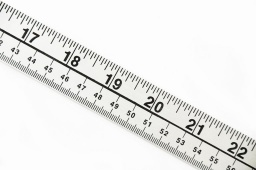Did you find an error or inaccuracy? Feel free to write us. Thank you!Tips to related online calculators
Need help to calculate sum, simplify or multiply fractions? Try our fraction calculator.
Do you want to convert length units?

## Related math problems and questions:

• Cutting boardFour pieces are measuring 29.5 cm. 19.62 cm, 32.49 cm, and 24.74 cm are cut from a board that is 200 cm long. Allowing 1 cm for total waste in cutting, what is the length of the remaining piece?
• PexesoDrawing paper has dimensions of 60cm and 840mm. Pupils have to divide it into squares so that they can make a pexeso. What dimension must squares have if their side is to be larger than 3cm and less than 10cm?
• Tartlets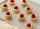After a special event, a caterer examined the leftovers on the serving table. She saw 10/11 of a tartlet with apples, 2/3 of a tartlet with strawberries, and 10/11 of a tartlet with raspberries. How many leftover tartlets did the caterer have?
• The heights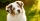The heights of some dogs are shown in the table. (1 cm = 10 mm) Dog Height Sam 280 mm Charlie 56 cm Gus 81.3 cm Buster 635 mm Max 38 cm Duke 61 cm Which list shows the heights of the dogs from tallest to shortest? A. Max, Charlie, Duke, Gus, Sam,Why does 1 3/4 + 2 9/10 equal 4.65? How do you solve this?
• Opposite numbers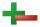Calculate opposite numbers (additive inverse) to given ones:
• Area and perimeter 2Find the area and the perimeter of a rectangle of length 45 1/2 cm and breadth 16 2/3 cm.
• The length 6The length of 12 pipes is 10 1/2 meters. (1) find the length of one pipe (2) also find the length of 7 pipes
• Pencil cut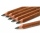Veronika cut 10cm pencil. Each turn, the pencil was reduced by 0.2mm. She turned the pencil 100 times. How many centimeters has a pencil now?
• PegsFrom two sticks 240 cm and 210 cm long, it is necessary to cut the longest possible pegs for flowers so that no residues remain. How many pegs will it be?
• Wood 11Father has 12 1/5 meters long wood. Then I cut the wood into two pieces. One part is 7 3/5 meters long. Calculate the length of the other wood?
• Square and circle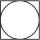Into square is an inscribed circle with diameter 10 cm. What is the difference between circumference square and circle?
• Rope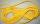Peter has a long rope 2 4/5 meter. Martin has a rope 20 cm longer. What distance ropes achieved if the boys link ropes?
• Bus vs train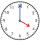Milada took the bus and the journey took 55 minutes. Jarmila was 1h 20 min by train. They arrived in Prague at the same time 10h45 min. At what time did each have to go out?
• My fatherMy father cut 78 slats on the fence. The shortest of them was 97 cm long, the longer one was 102 cm long. What was the total length of the slats in cm?
• Three rectangles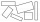Some wire is used to make 3 rectangles: A, B, and C. Rectangle B's dimensions are 3/5 cm larger than Rectangle A's dimensions, and Rectangle C's dimensions are again 3/5 cm larger than Rectangle B's dimensions. Rectangle A is 2 cm by 3 1/5 cm. What is the
• Frame image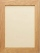Peter wants to put a picture in a frame. The picture is 7 3/5 cm wide. To fit in the picture frame cannot be more than 7 3/10 cm wide. How much should the image be trimmed?